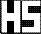Helbring Schültz: Publikationen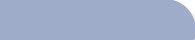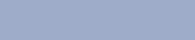[Download File as a Word-Document] Is 0! (read: "zero faculty") really 1? Reference-books describe the mathematical function "n-faculty" e.g., as quoted by Meyers Großem Rechen Duden in the following: "Faculty, n! (read: "n-faculty"). The product of the n numbers is called "n-faculty" for positive integer numbers. It is written n!=1 2 3 ... (n-1). 0! =1 is defined for n=0. Example: 1! =1=1       3!=1 2 3 = 6              2! =1 2 =2   ..................... n! = nn*e-n*sqrt(2*Pi*n) can be used approximately for big numbers (Stirling formula). 0!=1 is a convention without mathematical proof and thus is not conclusively a right statement. Following consideration makes the result 0 for 0! probable: In the equation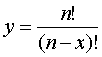x runs from 0 to n. Then a graph y over x is created with the gained value pairs. This graph shows at x=n an unsteadiness according to the up to now existing definition for 0!=1. On the other hand a continuous course would be guaranteed for 0!=0. Example for n=5 and x = 0,1,... 5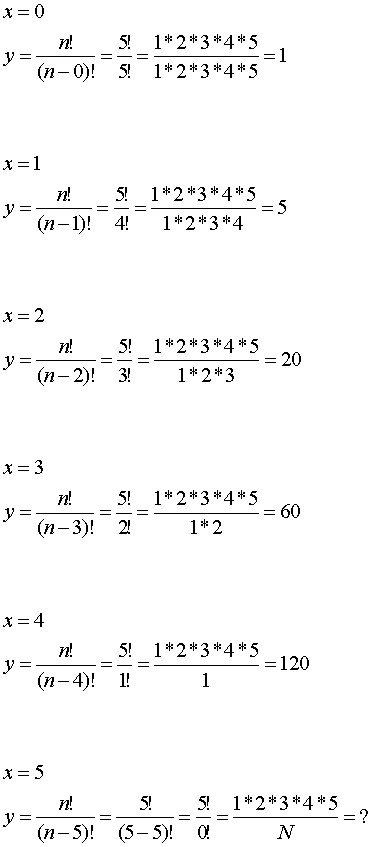N is set for 0!=1 in the denominator according to the valid definition. From this the same value 120 for y follows for the places x=4 and x=5, and causes in the further course of the graph an unsteadiness. This is shown by the dashed line. But if N is 0!=0 in the denominator, then the graph strives to §§§ in y direction, so that the given course of the graph is continued in the right direction. See graph. This behavior can definitely be reproduced for any number of n, e.g. n=8 with the place of unsteadiness at y=40.320, or for n=10 with the place of unsteadiness at y=3.628.800, and so on. Because of this consideration the statement 0!=0 may be more probable then the up to now definition of 0!=1. Thus the right answer of the question asked in the headline could be: 0!=1. Beyond it the definite result of the article above can be valued as true that 0!=0.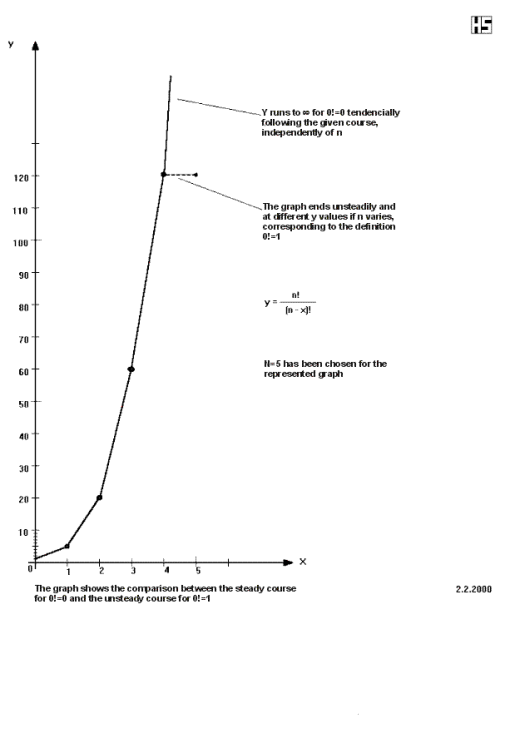Zum Seitenanfang springen